# Image Processing Filters#

Filters are mathematical operations that produce a new image out of one or more images. Pixel values between input and output images may differ.

import numpy as np

import matplotlib.pyplot as plt
from skimage import data
from skimage import filters
from skimage import morphology
from scipy.ndimage import convolve, gaussian_laplace
import stackview


To demonstrate what specific filters do, we start with a very simple image. It contains a lot of zeros and a single pixel with value 1 in the middle.

image1 = np.zeros((5, 5))
image1[2, 2] = 1
image1

array([[0., 0., 0., 0., 0.],
[0., 0., 0., 0., 0.],
[0., 0., 1., 0., 0.],
[0., 0., 0., 0., 0.],
[0., 0., 0., 0., 0.]])

plt.imshow(image1, cmap='gray')
plt.colorbar()

<matplotlib.colorbar.Colorbar at 0x162d12abe80>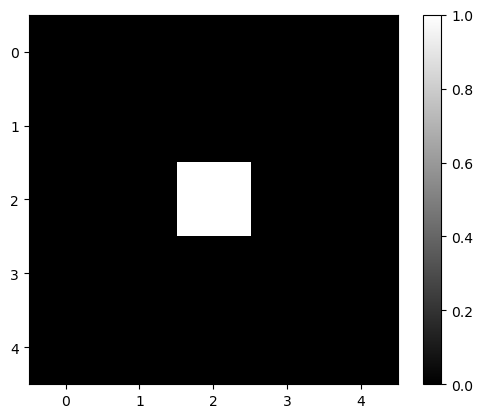## Gaussian kernel#

To apply a Gaussian blur to an image, we convolve it using a Gaussian kernel. The function gaussian in scikit-image can do this for us.

blurred = filters.gaussian(image1, sigma=1)
blurred

array([[0.00291504, 0.01306431, 0.02153941, 0.01306431, 0.00291504],
[0.01306431, 0.05855018, 0.09653293, 0.05855018, 0.01306431],
[0.02153941, 0.09653293, 0.15915589, 0.09653293, 0.02153941],
[0.01306431, 0.05855018, 0.09653293, 0.05855018, 0.01306431],
[0.00291504, 0.01306431, 0.02153941, 0.01306431, 0.00291504]])

plt.imshow(blurred, cmap='gray')
plt.colorbar()

<matplotlib.colorbar.Colorbar at 0x162d1363d00>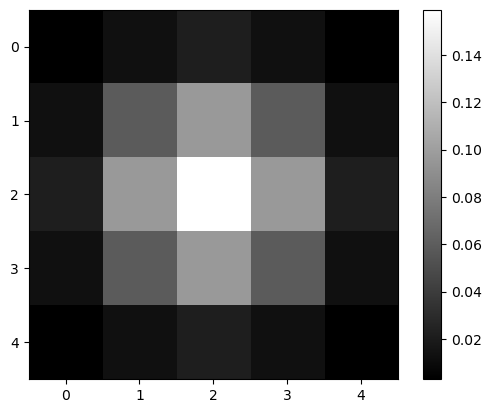## Laplacian#

Whenever you wonder what a filter might be doing, just create a simple test image and apply the filter to it.

image2 = np.zeros((9, 9))
image2[4, 4] = 1

plt.imshow(image2, cmap='gray')
plt.colorbar()

<matplotlib.colorbar.Colorbar at 0x162d1438fd0>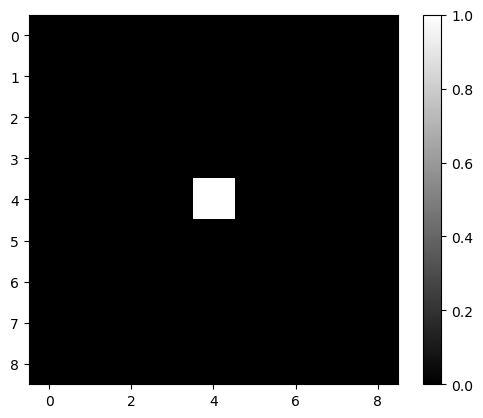mexican_hat = filters.laplace(image2)

plt.imshow(mexican_hat, cmap='gray')
plt.colorbar()

<matplotlib.colorbar.Colorbar at 0x162d1b312b0>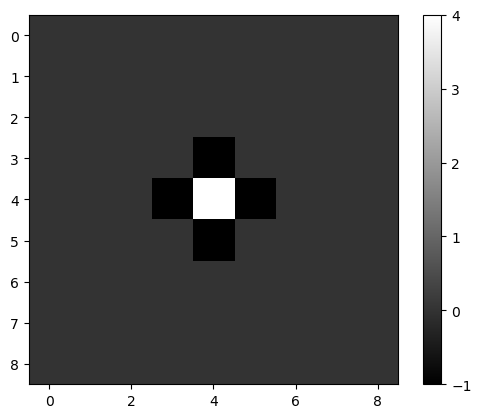## Laplacian of Gaussian#

We can also combine filters, e.g. using functions. If we apply a Gaussian filter to an image and a Laplacian afterwards, we have a filter doing the Laplacian of Gaussian (LoG) per definition.

def laplacian_of_gaussian(image, sigma):
"""
Applies a Gaussian kernel to an image and the Laplacian afterwards.
"""

# blur the image using a Gaussian kernel
intermediate_result = filters.gaussian(image, sigma)

# apply the mexican hat filter (Laplacian)
result = filters.laplace(intermediate_result)

return result

log_image1 = laplacian_of_gaussian(image2, sigma=1)

plt.imshow(log_image1, cmap='gray')
plt.colorbar()

<matplotlib.colorbar.Colorbar at 0x162d1bc5dc0>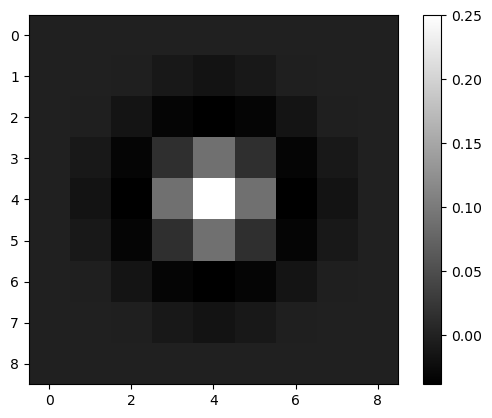## Interactive filter parameter tuning#

To understand better what filters are doing, it shall be recommended to apply them interactively. The following code will not render on github.com. You need to execute the notebook locally use this interactive user-interface.

image3 = imread('../../data/mitosis_mod.tif').astype(float)

stackview.interact(laplacian_of_gaussian, image3, zoom_factor=4)


## More filter examples#

We demonstrate some more typical filters using this nuclei example image.

plt.imshow(image3, cmap='gray')

<matplotlib.image.AxesImage at 0x162d1d5f1f0>## Denoising#

Common filters for denoising images are the mean filter, the median filter and the Gaussian filter.

denoised_mean = filters.rank.mean(image3.astype(np.uint8), morphology.disk(1))

plt.imshow(denoised_mean, cmap='gray')

<matplotlib.image.AxesImage at 0x162d1dc17c0>denoised_median = filters.median(image3, morphology.disk(1))

plt.imshow(denoised_median, cmap='gray')

<matplotlib.image.AxesImage at 0x162d1e4d220>denoised_median2 = filters.median(image3, morphology.disk(5))

plt.imshow(denoised_median2, cmap='gray')

<matplotlib.image.AxesImage at 0x162d30ab100>denoised_gaussian = filters.gaussian(image3, sigma=1)

plt.imshow(denoised_gaussian, cmap='gray')

<matplotlib.image.AxesImage at 0x162d2fa1970>We can also show these images side-by-side using matplotlib.

fig, axes = plt.subplots(1,3, figsize=(15,15))

axes.imshow(denoised_mean, cmap='gray')
axes.imshow(denoised_median, cmap='gray')
axes.imshow(denoised_gaussian, cmap='gray')

<matplotlib.image.AxesImage at 0x162d30dabb0>### Top-hat filtering / background removal#

top_hat = morphology.white_tophat(image3, morphology.disk(15))

plt.imshow(top_hat, cmap='gray')

<matplotlib.image.AxesImage at 0x162d3107e50>### Edge detection#

sobel = filters.sobel(image3)

plt.imshow(sobel, cmap='gray')

<matplotlib.image.AxesImage at 0x162d314a730>## Exercise#

Write a function that computes the Difference of Gaussian.

def difference_of_gaussian(image, sigma1, sigma2):

# enter code here


Use a simple function call to try out the function.

dog_image = difference_of_gaussian(image3, 1, 5)

plt.imshow(dog_image, cmap='gray')


Use the stackview library to play with it interactively.

stackview.interact(difference_of_gaussian, image3)